2022: SklogWiki celebrates 15 years on-line

# Difference between revisions of "Stirling's approximation"

James Stirling (1692-1770, Scotland)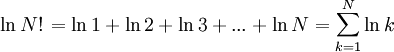$\left.\ln N!\right. = \ln 1 + \ln 2 + \ln 3 + ... + \ln N = \sum_{k=1}^N \ln k$

Because of [Euler-MacLaurin formula]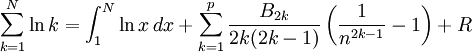$\sum_{k=1}^N \ln k=\int_1^N \ln x\,dx+\sum_{k=1}^p\frac{B_{2k}}{2k(2k-1)}\left(\frac{1}{n^{2k-1}}-1\right)+R$

where B1 = −1/2, B2 = 1/6, B3 = 0, B4 = −1/30, B5 = 0, B6 = 1/42, B7 = 0, B8 = −1/30, ... are the Bernoulli numbers, and R is an error term which is normally small for suitable values of p.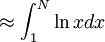$~\approx \int_1^N \ln x dx$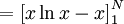$~= \left[ x \ln x - x \right]_1^N$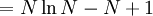$~= N \ln N -N +1$

Thus, for large N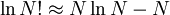$\ln N! \approx N \ln N -N$